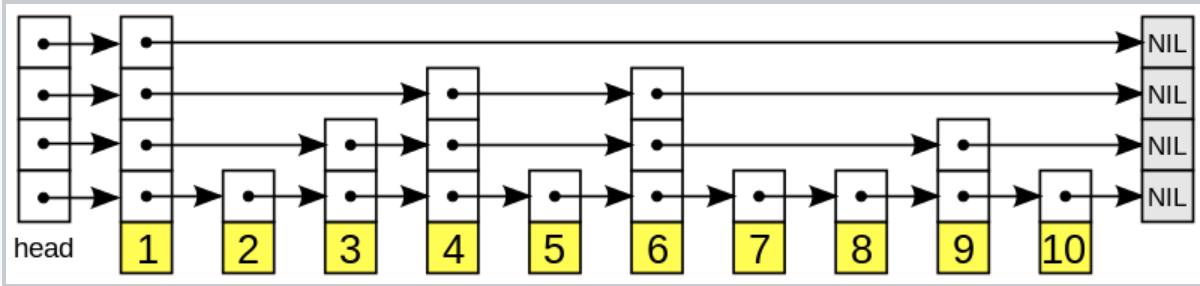# Skip List Implementation with C++

In computer science, a skip list is a data structure that allows O(log(n)) search complexity as well as O(log(n)) insertion complexity within an ordered sequence of n elements.

You can read more about it on WikipediaIn this post I’d like to jot down the notes that prove the asymptotic runtime of the search and insertion algorithm.

You can also watch MIT’s course video on SkipList.

## Search Cost

The elements in L1 are assumed to be interspersed equally and therefore with 2 linked lists L0 and L1, the search cost approx. equals

Because in the worst case you’ll have to traverse for |L1|-1 element and move downwards, and then traverse the rest of L0. Since L1 is interspersed equally, the remaining L0 to traverse is at most

In order to minimise the search cost, we apply second partial derivative test to learn how many elements there should be in L1. let the cardinality of L1 be x and cardinality of L0 be y, we have

let $\frac{\partial f(x,y)}{\partial x}= 1-\frac{y}{x^2} = 0$, we have

Second partial derivative test: $H = \frac{\partial f(x,y)}{\partial x^2}\frac{\partial f(x,y)}{\partial y^2} - \frac{\partial f(x,y)}{\partial y^2}$

Since we have identified critical points with $\frac{\partial f(x,y)}{\partial x}=0$.

Let’s test whether points on $y = x^2$ represents minima of maxima.

We use a random point on $y = x^2$ as a test, point (2,4)

$H = \frac{\partial f(x,y)}{\partial x^2}\frac{\partial f(x,y)}{\partial y^2} - \frac{\partial f(x,y)}{\partial y^2}$

since H > 0, the test point is either maximum or minimum.

Then we check $\frac{\partial f(x,y)}{\partial x^2}(2,4)=1>0$, we conclude that the point on $y = x^2$ represents minima.

Since we have

For a skip list with 2 link lists, in order to minimize the search cost we need to have the upper level list to have

We conclude:

The search cost of a skip list with 2 linked lists is O(sqrt(n))

We can generalise the conclusion with:

2 linked lists, search cost $= 2 \times n^{\frac{1}{2}}$
3 linked lists, search cost $= 3 \times n^{\frac{1}{3}}$
4 linked lists, search cost $= 4 \times n^{\frac{1}{4}}$

$\log_2{n}$ linked lists, search cost = $\log_2{n} \times n^{\frac{1}{\log_2{n}}}$

Since $\frac{1}{log_2{n}} = \frac{\log_2{2}}{\log_2{n}} = \log_n{2}$ , we have search cost $= 2 \times \log_2{n}$

We generalise the conclusion:

The search cost of a skip list with log(n) linked lists (i.e. log(n) levels) is O(log(n))

## Achieving log(n) Levels With Coin Toss

We want to build a skip list from null and therefore when inserting we need to decide for how many levels this new element needs to be in. With coin tosses, we check for how many heads we have got until we get the first tail. This method would achieve log(n) levels for a n element skip list with high probability.

Lemma: The number of levels in a n-element skip list is O(log(n)) with high probability.

With O(log(n)), we know the number of levels in a n-element skip list should be $c \times \log_2{n} + c$ becoz big O is the upper bound, and for simplicity we ignore the constant.

We define with high probability as $1 - \frac{1}{n^\alpha}\\$

It means that when n grows, $\frac{1}{n^\alpha}$ will shrink towards zero and $1-\frac{1}{n^\alpha} \approx 1$.

Proof:

let failure probability be P(levels not <= c*lgg(n))
= P(levels > c*log(n))
= P(there exists some elements such that their levels > c*log(n))

<= n * P(element X has levels > c*log(n))


The last inequality is true because of Boole’s inequality, or union bound.

We can then calculate

## Seach, With High Probability

theorem: any search in an n-element skip list costs O(log(n)) with high probability.

Consider the reverse of the search algorithm:

with each successful search, you start at the found node:
while current node is not sentinel:
while current node allows move up:
go up
move left


For each move up or left, the probability is 1/2 because if you are allowed to move upward, it means at the point of insertion of this element, you successfully tossed a head to get a promotion to the next level.

Therefore the number of up moves < # of levels <= c * log(n). Total number of moves = total moves until you reach c*log(n) up moves.

Claim: total # of moves = # of coin flips until c*log(n) heads are obtained = O(log(n)) with high probability

Proof:
let Y be a random variable representing
the total number of tails in
a series of m independent coin flips
where each flip has a probability p of coming up heads.

then for all r>0 we have (Chernoff's Bound)


$P( Y >= E[Y]+r ) \leq e^{ \frac{-2(r^2)}{m}}\\$

Chernoff Bound tells us the probability of Y being larger than the expected value by r

Cheers!